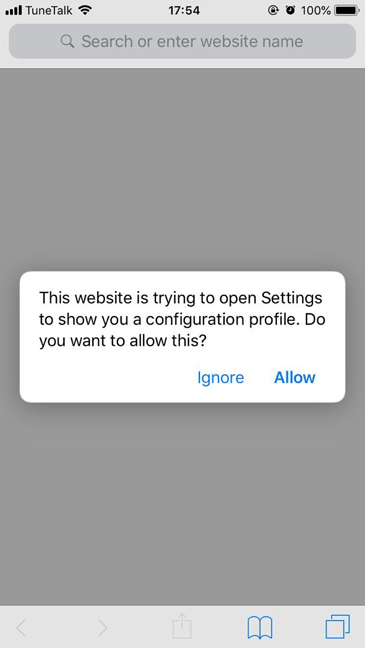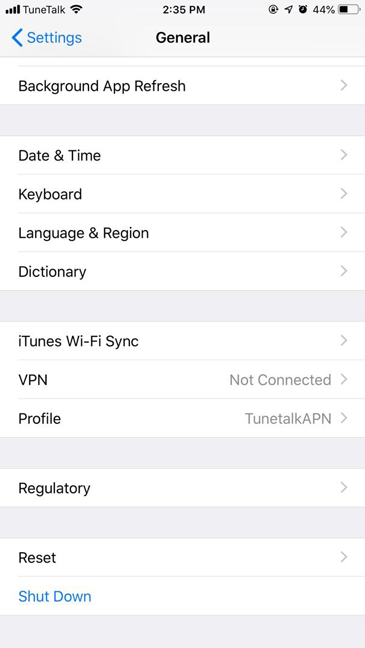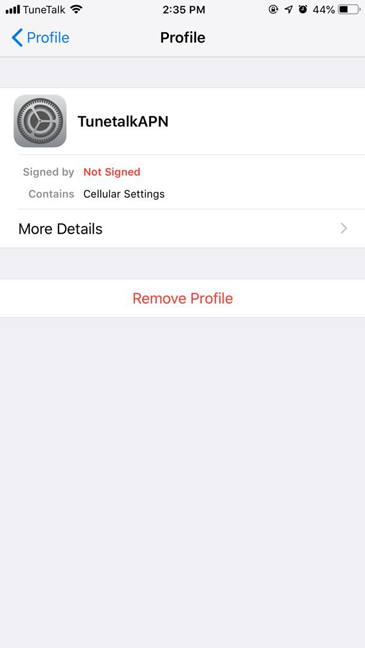Having problems with your data connection in or out of Malaysia?

Please select your desired mobile operating system and follow the instructions to manually configure your device. Do note that the settings may vary depending on your devices.

Version 12.1.1 and above

STEP 1
*Please use the default Safari browser to download the file.STEP 2
Allow your Safari browser to open the configuration profile.STEP 3
Install the TunetalkAPN.STEP 4
Sit back and let us do the rest for you

To make sure you have installed the correct configuration file, here is how you can check your profileSTEP 1
Go to Settings > General > Profile > TunetalkAPNSTEP 2
Tap on 'More Details' to view the configuration details.STEP 3
Make sure the correct APN as below:

Attach APN: tunetalk
APN: tunetalk.

Earlier Version

STEP 1

Go to Setting > Cellular > Enabled Cellular Data

STEP 2

Choose Cellular Data Network

STEP 3

Cellular Data Settings > APN: tunetalk (leave other fields blank as default)

STEP 4

Switch OFF your device > Turn ON your device to start browsing the internet!

*For users on roaming, please ensure “Data Roaming” is enabled.

STEP 1

Go to Setting > More networks > Mobile Networks > Tick Mobile Data

STEP 2

Select Access Point Names > Option

STEP 3

New APN > APN: tunetalk Autentication Type: PAP (leave other fields blank as default) > Save > Tune Talk Internet

STEP 4

Switch OFF your device > Turn ON your device to start browsing the internet!

*For users on roaming, please ensure “Data Roaming” is enabled.

Response time: 0.57553219795227
1. select * from `rainlab_translate_attributes` where `locale` = ? and `model_id` = ? and `model_type` = ? limit 1
2. select * from `rainlab_translate_attributes` where `locale` = ? and `model_id` = ? and `model_type` = ? limit 1
3. select * from `rainlab_translate_attributes` where `locale` = ? and `model_id` = ? and `model_type` = ? limit 1
4. select * from `rainlab_translate_attributes` where `locale` = ? and `model_id` = ? and `model_type` = ? limit 1
5. select * from `rainlab_translate_attributes` where `locale` = ? and `model_id` = ? and `model_type` = ? limit 1
6. select * from `rainlab_translate_attributes` where `locale` = ? and `model_id` = ? and `model_type` = ? limit 1
7. select * from `rainlab_translate_attributes` where `locale` = ? and `model_id` = ? and `model_type` = ? limit 1
8. select * from `rainlab_translate_attributes` where `locale` = ? and `model_id` = ? and `model_type` = ? limit 1
9. select * from `rainlab_translate_attributes` where `locale` = ? and `model_id` = ? and `model_type` = ? limit 1
10. select * from `rainlab_translate_attributes` where `locale` = ? and `model_id` = ? and `model_type` = ? limit 1
11. select * from `rainlab_translate_attributes` where `locale` = ? and `model_id` = ? and `model_type` = ? limit 1
12. select * from `rainlab_translate_attributes` where `locale` = ? and `model_id` = ? and `model_type` = ? limit 1
13. select * from `rainlab_translate_attributes` where `locale` = ? and `model_id` = ? and `model_type` = ? limit 1
14. select * from `rainlab_translate_attributes` where `locale` = ? and `model_id` = ? and `model_type` = ? limit 1
15. select * from `rainlab_translate_attributes` where `locale` = ? and `model_id` = ? and `model_type` = ? limit 1
16. select * from `rainlab_translate_attributes` where `locale` = ? and `model_id` = ? and `model_type` = ? limit 1
17. select * from `rainlab_translate_attributes` where `locale` = ? and `model_id` = ? and `model_type` = ? limit 1
18. select * from `rainlab_translate_attributes` where `locale` = ? and `model_id` = ? and `model_type` = ? limit 1
19. select * from `rainlab_translate_attributes` where `locale` = ? and `model_id` = ? and `model_type` = ? limit 1
20. select * from `rainlab_translate_attributes` where `locale` = ? and `model_id` = ? and `model_type` = ? limit 1
21. select * from `rainlab_translate_attributes` where `locale` = ? and `model_id` = ? and `model_type` = ? limit 1
22. select * from `rainlab_translate_attributes` where `locale` = ? and `model_id` = ? and `model_type` = ? limit 1
23. select * from `rainlab_translate_attributes` where `locale` = ? and `model_id` = ? and `model_type` = ? limit 1
24. select * from `rainlab_translate_attributes` where `locale` = ? and `model_id` = ? and `model_type` = ? limit 1
25. select * from `rainlab_translate_attributes` where `locale` = ? and `model_id` = ? and `model_type` = ? limit 1
26. select * from `rainlab_translate_attributes` where `locale` = ? and `model_id` = ? and `model_type` = ? limit 1
27. select * from `rainlab_translate_attributes` where `locale` = ? and `model_id` = ? and `model_type` = ? limit 1
28. select * from `rainlab_translate_attributes` where `locale` = ? and `model_id` = ? and `model_type` = ? limit 1
29. select * from `rainlab_translate_attributes` where `locale` = ? and `model_id` = ? and `model_type` = ? limit 1
30. select * from `rainlab_translate_attributes` where `locale` = ? and `model_id` = ? and `model_type` = ? limit 1
31. select * from `rainlab_translate_attributes` where `locale` = ? and `model_id` = ? and `model_type` = ? limit 1
32. select * from `rainlab_translate_attributes` where `locale` = ? and `model_id` = ? and `model_type` = ? limit 1
33. select * from `rainlab_translate_attributes` where `locale` = ? and `model_id` = ? and `model_type` = ? limit 1
34. select * from `rainlab_translate_attributes` where `locale` = ? and `model_id` = ? and `model_type` = ? limit 1
35. select * from `rainlab_translate_attributes` where `locale` = ? and `model_id` = ? and `model_type` = ? limit 1
36. select * from `rainlab_translate_attributes` where `locale` = ? and `model_id` = ? and `model_type` = ? limit 1
37. select * from `rainlab_translate_attributes` where `locale` = ? and `model_id` = ? and `model_type` = ? limit 1
38. select * from `rainlab_translate_attributes` where `locale` = ? and `model_id` = ? and `model_type` = ? limit 1
39. select * from `rainlab_translate_attributes` where `locale` = ? and `model_id` = ? and `model_type` = ? limit 1
40. select * from `rainlab_translate_attributes` where `locale` = ? and `model_id` = ? and `model_type` = ? limit 1
41. select * from `rainlab_translate_attributes` where `locale` = ? and `model_id` = ? and `model_type` = ? limit 1
42. select * from `rainlab_translate_attributes` where `locale` = ? and `model_id` = ? and `model_type` = ? limit 1
43. select * from `rainlab_translate_attributes` where `locale` = ? and `model_id` = ? and `model_type` = ? limit 1
44. select * from `rainlab_translate_attributes` where `locale` = ? and `model_id` = ? and `model_type` = ? limit 1
45. select * from `rainlab_translate_attributes` where `locale` = ? and `model_id` = ? and `model_type` = ? limit 1
46. select * from `rainlab_translate_attributes` where `locale` = ? and `model_id` = ? and `model_type` = ? limit 1
47. select * from `rainlab_translate_attributes` where `locale` = ? and `model_id` = ? and `model_type` = ? limit 1
48. select * from `rainlab_translate_attributes` where `locale` = ? and `model_id` = ? and `model_type` = ? limit 1
49. select * from `rainlab_translate_attributes` where `locale` = ? and `model_id` = ? and `model_type` = ? limit 1
50. select * from `rainlab_translate_attributes` where `locale` = ? and `model_id` = ? and `model_type` = ? limit 1
51. select * from `rainlab_translate_attributes` where `locale` = ? and `model_id` = ? and `model_type` = ? limit 1
52. select * from `rainlab_translate_attributes` where `locale` = ? and `model_id` = ? and `model_type` = ? limit 1
53. select * from `rainlab_translate_attributes` where `locale` = ? and `model_id` = ? and `model_type` = ? limit 1
54. select * from `rainlab_translate_attributes` where `locale` = ? and `model_id` = ? and `model_type` = ? limit 1
55. select * from `rainlab_translate_attributes` where `locale` = ? and `model_id` = ? and `model_type` = ? limit 1
56. select * from `rainlab_translate_attributes` where `locale` = ? and `model_id` = ? and `model_type` = ? limit 1
57. select * from `rainlab_translate_attributes` where `locale` = ? and `model_id` = ? and `model_type` = ? limit 1
58. select * from `rainlab_translate_attributes` where `locale` = ? and `model_id` = ? and `model_type` = ? limit 1
59. select * from `rainlab_translate_attributes` where `locale` = ? and `model_id` = ? and `model_type` = ? limit 1
60. select * from `rainlab_translate_attributes` where `locale` = ? and `model_id` = ? and `model_type` = ? limit 1
61. select * from `rainlab_translate_attributes` where `locale` = ? and `model_id` = ? and `model_type` = ? limit 1
62. select * from `rainlab_translate_attributes` where `locale` = ? and `model_id` = ? and `model_type` = ? limit 1
63. select * from `rainlab_translate_attributes` where `locale` = ? and `model_id` = ? and `model_type` = ? limit 1
64. select * from `rainlab_translate_attributes` where `locale` = ? and `model_id` = ? and `model_type` = ? limit 1
65. select * from `rainlab_translate_attributes` where `locale` = ? and `model_id` = ? and `model_type` = ? limit 1
66. select * from `rainlab_translate_attributes` where `locale` = ? and `model_id` = ? and `model_type` = ? limit 1
67. select * from `rainlab_translate_attributes` where `locale` = ? and `model_id` = ? and `model_type` = ? limit 1
68. select * from `rainlab_translate_attributes` where `locale` = ? and `model_id` = ? and `model_type` = ? limit 1
69. select * from `rainlab_translate_attributes` where `locale` = ? and `model_id` = ? and `model_type` = ? limit 1
70. select * from `rainlab_translate_locales` where `code` = ? limit 1
71. select * from `rainlab_translate_attributes` where `locale` = ? and `model_id` = ? and `model_type` = ? limit 1
72. select * from `rainlab_translate_attributes` where `locale` = ? and `model_id` = ? and `model_type` = ? limit 1
73. select * from `rainlab_translate_attributes` where `locale` = ? and `model_id` = ? and `model_type` = ? limit 1
74. select * from `rainlab_translate_attributes` where `locale` = ? and `model_id` = ? and `model_type` = ? limit 1
75. select * from `rainlab_translate_attributes` where `locale` = ? and `model_id` = ? and `model_type` = ? limit 1
76. select * from `rainlab_translate_attributes` where `locale` = ? and `model_id` = ? and `model_type` = ? limit 1
77. select * from `rainlab_translate_attributes` where `locale` = ? and `model_id` = ? and `model_type` = ? limit 1
78. select * from `rainlab_translate_attributes` where `locale` = ? and `model_id` = ? and `model_type` = ? limit 1